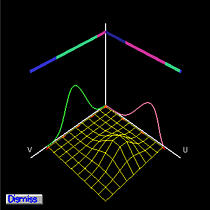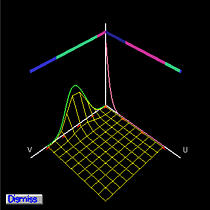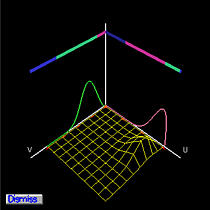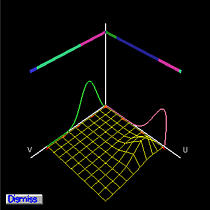# The Partition of Unity WindowThe Partition of Unity window provides a user with two important pieces of information. You can activate this window by selecting Windows, followed by Show Partition of Unity Window. When this window appears, if there is no control point selected (using the right mouse button), it contains nothing but the u- and v- axes.

Once a control point is selected, the Partition of Unity window shows the coefficient of the selected control point. Since this is a tensor product surface, the coefficient of a control point is the product of two basis functions. The basis functions are displayed on the u-axis and the v-axis, and their product, the coefficient of the selected control point, is shown in wireframe. The following figures show the coefficients of two different selected control points.The actual value of the coefficient depends on the current values of u and v. Once a u (resp., v) is given, all basis functions at this u (resp., v) partition the interval [0,1] into shorter interval. The lengths of these subintervals are the weights contributing to control points. The top two color bars give the way of partition of unity. The following figures show the partitions of unity at two different (u,v)'s. Please note that the basis functions of the selected control point are the same; but as (u,v) changes (by dragging the uv-indicator), the way of partition of unity changes accordingly. As a result, the coefficient (i.e., product of the basis functions) will be different for different (u,v).If a surface is subdivided, one can click the domain of a subpatch to move to that surface. Then, selecting a control point of that patch will cause the partition of unity of that patch to appear. Please note that the basis function being displayed will have a smaller domain, because it is for a subpatch.